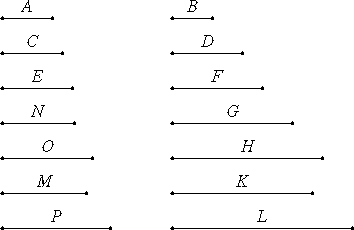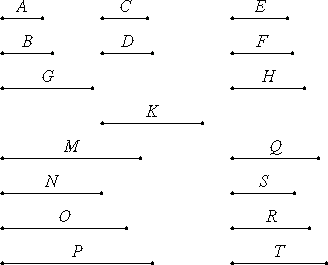# Proposition 4

Given as many ratios as we please in least numbers, to find numbers in continued proportion which are the least in the given ratios.

Let the given ratios in least numbers be that of A to B, that of C to D, and that of E to F.

It is required to find numbers in continued proportion which are the least that are in the ratio of A to B, in the ratio of C to D, and in the ratio of E to F.VII.34

Take G, the least number measured by B and C.

Let A measure H as many times as B measures G, and let D measure K as many times as C measures G.

Now E either measures or does not measure K.

First, let it measure it. Let K measure L as many times as E measures K.

Now, since A measures H the same number of times that B measures G, therefore A is to B as H is to G.

For the same reason C is to D as G is to K, and E is to F as K is to L. Therefore H, G, K, and L are continuously proportional in the ratio of A to B, in the ratio of C to D, and in the ratio of E to F.

I say next that they are also the least that have this property.

If H, G, K, and L are not the least numbers continuously proportional in the ratios of A to B, of C to D, and of E to F, let them be N, O, M, and P.

VII.20

Then since A is to B as N is to O, while A and B are least, and the least numbers measure those which have the same ratio the same number of times, the greater the greater and the less the less, that is, the antecedent the antecedent and the consequent the consequent, therefore B measures O.

VII.35

For the same reason C also measures O. Therefore B and C measure O. Therefore the least number measured by B and C also measures O.

But G is the least number measured by B and C, therefore G measures O, the greater the less, which is impossible. Therefore there are no numbers less than H, G, K, and L which are continuously in the ratio of A to B, of C to D, and of E to F.

Next, let E not measure K.

Take M, the least number measured by E and K. Let H and G measure N and O as many times as K measures M, respectively, and let F measure P as many times as E measures M.Since H measures N the same number of times that G measures O, therefore H is to G as N is to O. But H is to G as A is to B, therefore A is to B as is N is to O. For the same reason C is to D as is O is to M.

Again, since E measures M the same number of times that F measures P, therefore E is to F as M is to P. Therefore N, O, M, and P are continuously proportional in the ratios of A to B, of C to D, and of E to F.

I say next that they are also the least that are in the ratios A, B, C, D, E, and F.

If not, there are numbers less than N, O, M, and P continuously proportional in the ratios A, B, C, D, E, and F. Let them be Q, R, S, and T.

VII.20

Now since Q is to R as A is to B, while A and B are least, and the least numbers measure those which have the same ratio with them the same number of times, the antecedent the antecedent and the consequent the consequent, therefore B measures R. For the same reason C also measures R, therefore B and C measure R.

VII.35

Therefore the least number measured by B and C also measures R. But G is the least number measured by B and C, therefore G measures R.

VII.13

And G is to R as K is to S, therefore K also measures S.

But E also measures S. Therefore E and K measure S.

Therefore the least number measured by E and K also measures S. But M is the least number measured by E and K, therefore M measures S, the greater the less, which is impossible.

VII.35

Therefore there are no numbers less than N, O, M, and P continuously proportional in the ratios of A to B, of C to D, and of E to F. Therefore N, O, M, and P are the least numbers continuously proportional in the ratios A, B, C, D, E, and F.

Q.E.D.

## Guide

This is a generalization of VIII.2 to a more general concept of continued proportion. In this proposition we consider continued proportions having ratios that aren’t necessarily constant. These, perhaps, should be called continued ratios. For example, the continued ratio 5 : 10 : 20 has a constant ratio of 1 : 2, but the continued ratio 5 : 10 : 30 does not; its first ratio is 1 : 2 while its second ratio is 1 : 3. Note that the continued ratio 5 : 10 : 30 is not the least with those given ratios since 1 : 2 : 6 is smaller with the same ratios of 1 : 2 and 1 : 3.

The problem here is to construct the smallest continued ratio having the specified ratios.

An examination of the proof shows that Euclid has a general process to attach two continued proportions into one long one with with the same ratios. Take, for example, the problem of placing the continued ratio 3 : 7 : 2 : 6 in front of the continued ratio 10 : 4 : 5 to make a seven-term continued ratio where the first four terms have the ratio 3 : 7 : 2 : 6 and the last three terms have the ratio 10 : 4 : 5. The resulting seven-term ratio should be least with the given ratios. The problem is that the last term of the first ratio, 6, does not equal the first term of the second ratio, 10. The solution is to increase the numbers in each ratio to match these numbers. Since 30 = LCM(6, 10), that can be done by multiplying each of the terms of the first ratio by 5 and each of the terms of the second ratio by 3. The resulting ratios, 15 : 35 : 10 : 30 and 30 : 12 : 15 can then be merged into the desired ratio 15 : 35 : 10 : 30 : 12 : 15.

#### Outline of the proof

We start with three given ratios a : b, c : d, and e : f, all in lowest terms. First, the two ratios a : b and c : d are merged into a three-term ratio h : g : k so that h : g = a : b and g : k = c : d. These are defined equationally as g = LCM(b, c), h = (g/b)a, and k = (g/c)d. Then h : g : k has the proper ratios.

Next, the three-term ratio h : g : k is merged with the ratio e : f to get a four-term ratio n : o : m : p so that n : o = a : b, o : m = c : d, and m : p = c : d. These are defined equationally as m = LCM(e, k), n = (m/k)h, and o = (m/k)g, and p = (m/e)f. Again, n : o : m : p has the proper ratios.

The bulk of the proof consists of showing that the resulting ratio n : o : m : p is the least with the given ratios.

#### Use of this proposition

This proposition is used in the next one and in proposition X.12.